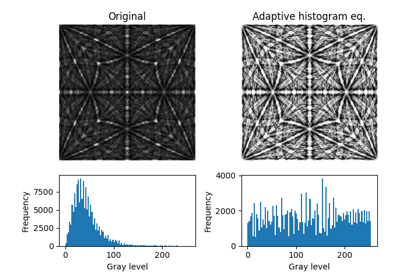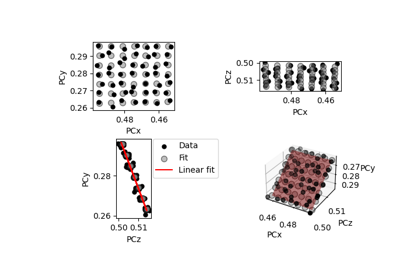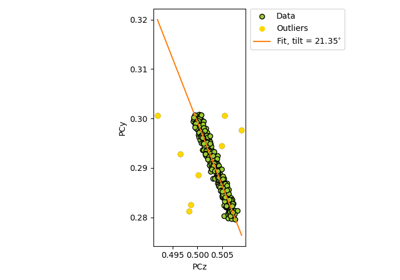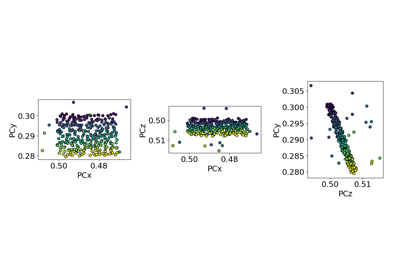# EBSDDetector#

class kikuchipy.detectors.EBSDDetector(shape: Tuple[int, int] = (1, 1), px_size: float = 1, binning: int = 1, tilt: float = 0, azimuthal: float = 0, sample_tilt: float = 70, pc: = (0.5, 0.5, 0.5), convention: = None)[source]#

Bases: object

An EBSD detector class storing its shape, pixel size, binning factor, detector tilt, sample tilt and projection center (PC) per pattern. Given one or multiple PCs, the detector’s gnomonic coordinates are calculated. Uses of these include projecting Kikuchi bands, given a unit cell, unit cell orientation and family of planes, onto the detector.

Calculation of gnomonic coordinates is based on the work by Aimo Winkelmann in the supplementary material to .

Parameters:
shape

Number of detector rows and columns in pixels. Default is (1, 1).

px_size

Size of unbinned detector pixel in um, assuming a square pixel shape. Default is 1.

binning

Detector binning, i.e. how many pixels are binned into one. Default is 1, i.e. no binning.

tilt

Detector tilt from horizontal in degrees. Default is 0.

azimuthal

Sample tilt about the sample RD (downwards) axis. A positive angle means the sample normal moves towards the right looking from the sample to the detector. Default is 0.

sample_tilt

Sample tilt from horizontal in degrees. Default is 70.

pc

X, Y and Z coordinates of the projection/pattern centers (PCs), describing the location of the beam on the sample measured relative to the detection screen. PCs are stored and used in Bruker’s convention. See Notes for the definition and conversions between conventions. If multiple PCs are passed, they are assumed to be on the form [[x0, y0, z0], [x1, y1, z1], …]. Default is [0.5, 0.5, 0.5].

convention

Convention of input PC, to determine which conversion to Bruker’s definition to use. If not given, Bruker’s convention is assumed. Options are “tsl”/”edax”/”amatek”, “oxford”/”aztec”, “bruker”, “emsoft”, “emsoft4”, and “emsoft5”. “emsoft” and “emsoft5” is the same convention. See Notes for conversions between conventions.

Notes

The pattern on the detector is always viewed from the detector towards the sample. Pattern width and height is here given as $$N_x$$ and $$N_y$$ (possibly binned). PCs are stored and used in Bruker’s convention.

The Bruker PC coordinates $$(x_B^*, y_B^*, z_B^*)$$ are defined in fractions of $$N_x$$, $$N_y$$, and $$N_y$$, respectively, with $$x_B^*$$ and $$y_B^*$$ defined with respect to the upper left corner of the detector. These coordinates are used internally, called $$(PC_x, PC_y, PC_z)$$ in the rest of the documentation when there is no reference to Bruker specifically.

The EDAX TSL PC coordinates $$(x_T^*, y_T^*, z_T^*)$$ and Oxford Instruments PC coordinates $$(x_O^*, y_O^*, z_O^*)$$ are identical and defined in fractions of $$N_x$$ with respect to the lower left corner of the detector.

The EMsoft PC coordinates $$(x_{pc}, y_{pc})$$ are defined as number of pixels (subpixel accuracy) with respect to the center of the detector, with $$x_{pc}$$ towards the right and $$y_{pc}$$ upwards. The final PC coordinate $$L$$ is the detector distance in microns. Note that prior to EMsoft v5.0, $$x_{pc}$$ was defined towards the left.

Given these definitions, the following is the conversion from TSL/Oxford to Bruker

$\begin{split}x_B^* &= x_T^*,\\ y_B^* &= 1 - \frac{N_x}{N_y} y_T^*,\\ z_B^* &= \frac{N_x}{N_y} z_T^*.\end{split}$

The conversion from EMsoft to Bruker is given as

$\begin{split}x_B^* &= \frac{1}{2} - \frac{x_{pc}}{N_x b},\\ y_B^* &= \frac{1}{2} - \frac{y_{pc}}{N_y b},\\ z_B^* &= \frac{L}{N_y b \delta},\end{split}$

where $$\delta$$ is the unbinned detector pixel size in microns, and $$b$$ is the binning factor.

Examples

Create an EBSD detector and plot the PC on top of a pattern

>>> import numpy as np
>>> import kikuchipy as kp
>>> det = kp.detectors.EBSDDetector(
...     shape=(60, 60),
...     pc=np.ones((10, 20, 3)) * (0.421, 0.779, 0.505),
...     convention="edax",
...     px_size=70,
...     binning=8,
...     tilt=5,
...     sample_tilt=70,
... )
>>> det
EBSDDetector (60, 60), px_size 70 um, binning 8, tilt 5, azimuthal 0, pc (0.421, 0.221, 0.505)
(10, 20)
>>> det.bounds
array([ 0, 59,  0, 59])
>>> det.gnomonic_bounds[0, 0]
array([-0.83366337,  1.14653465, -1.54257426,  0.43762376])
>>> s = kp.data.nickel_ebsd_small()
>>> det.plot(
...     pattern=s.inav[0, 0].data,
...     coordinates="gnomonic",
...     draw_gnomonic_circles=True
... )


Attributes

 EBSDDetector.aspect_ratio Return the number of detector columns divided by rows. EBSDDetector.bounds Return the detector bounds [x0, x1, y0, y1] in pixel coordinates. EBSDDetector.gnomonic_bounds Return the detector bounds [x0, x1, y0, y1] in gnomonic coordinates. EBSDDetector.height Return the detector height in microns. EBSDDetector.navigation_dimension Return the number of navigation dimensions of the projection center array (a maximum of 2). EBSDDetector.navigation_shape Return or set the navigation shape of the projection center array. EBSDDetector.navigation_size Return the number of projection centers. EBSDDetector.ncols Return the number of detector pixel columns. EBSDDetector.nrows Return the number of detector pixel rows. EBSDDetector.pc Return or set all projection center coordinates. EBSDDetector.pc_average Return the overall average projection center. EBSDDetector.pc_flattened Return flattened array of projection center coordinates of shape (navigation_size, 3). EBSDDetector.pcx Return or set the projection center x coordinates. EBSDDetector.pcy Return or set the projection center y coordinates. EBSDDetector.pcz Return or set the projection center z coordinates. EBSDDetector.px_size_binned Return the binned pixel size in microns. EBSDDetector.r_max Return the maximum distance from PC to detector edge in gnomonic coordinates. EBSDDetector.size Return the number of detector pixels. EBSDDetector.specimen_scintillator_distance Return the specimen to scintillator distance, known in EMsoft as $$L$$. EBSDDetector.unbinned_shape Return the unbinned detector shape in pixels. EBSDDetector.width Return the detector width in microns. EBSDDetector.x_max Return the right bound of detector in gnomonic coordinates. EBSDDetector.x_min Return the left bound of detector in gnomonic coordinates. EBSDDetector.x_range Return the x detector limits in gnomonic coordinates. EBSDDetector.x_scale Return the width of a pixel in gnomonic coordinates. EBSDDetector.y_max Return the bottom bound of detector in gnomonic coordinates. EBSDDetector.y_min Return the top bound of detector in gnomonic coordinates. EBSDDetector.y_range Return the y detector limits in gnomonic coordinates. EBSDDetector.y_scale Return the height of a pixel in gnomonic coordinates.

Methods

 EBSDDetector.crop(extent) Return a new detector with its shape cropped and pc values updated accordingly. Return a deep copy using copy.deepcopy(). Estimate the tilt about the detector $$X_d$$ axis. EBSDDetector.estimate_xtilt_ztilt([degrees, ...]) Estimate the tilts about the detector $$X_d$$ and $$Z_d$$ axes. EBSDDetector.extrapolate_pc(pc_indices, ...) Return a new detector with projection centers (PCs) in a 2D map extrapolated from an average PC. EBSDDetector.fit_pc(pc_indices, map_indices) Return a new detector with interpolated projection centers (PCs) for all points in a map by fitting a plane to pc . EBSDDetector.get_indexer(phase_list, **kwargs) Return a PyEBSDIndex EBSD indexer. EBSDDetector.load(fname) Return an EBSD detector loaded from a text file saved with save(). Return PC in the Bruker convention, given in the class description. EBSDDetector.pc_emsoft([version]) Return PC in the EMsoft convention. Return PC in the Oxford convention. Return PC in the EDAX TSL convention. EBSDDetector.plot([coordinates, show_pc, ...]) Plot the detector screen viewed from the detector towards the sample. EBSDDetector.plot_pc([mode, return_figure, ...]) Plot all projection centers (PCs). EBSDDetector.save(filename[, convention]) Save detector in a text file with projection centers (PCs) in the given convention.

## Examples using EBSDDetector#Fit a plane to selected projection centers

Fit a plane to selected projection centersEstimate tilt about the detector x axis

Estimate tilt about the detector x axisEstimate tilts about the detector x and z axis

Estimate tilts about the detector x and z axis